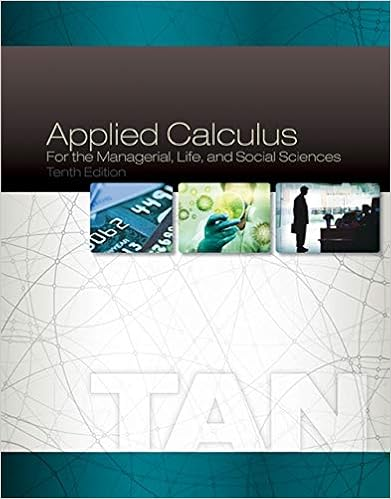# ECON2301 Quiz 3 Ch. 8-9 Answers - PRINCIPLES OF...

• Test Prep
• 6
• 100% (13) 13 out of 13 people found this document helpful

This preview shows page 1 - 3 out of 6 pages.

##### We have textbook solutions for you!
The document you are viewing contains questions related to this textbook.The document you are viewing contains questions related to this textbook.
Chapter 6 / Exercise 2
Applied Calculus for the Managerial, Life, and Social Sciences
TanExpert Verified
PRINCIPLES OF MACROECONOMICS QUIZ #3 – COVERING CHAPTERS 8 AND 9 ANSWERS 1.What is the relationship between consumption and the following economic variables: household income, wealth, household's expectations about the future, and interest rates?
2.Draw a graph of the consumption function and explain what the vertical intercept means and the slope. Make sure to label both axes.
3.Explain any differences between actual investment and planned investment. Also, is it possible for actual investment to be greater than planned investment? If so, explain.
##### We have textbook solutions for you!
The document you are viewing contains questions related to this textbook.The document you are viewing contains questions related to this textbook.
Chapter 6 / Exercise 2
Applied Calculus for the Managerial, Life, and Social Sciences
TanExpert Verified
4.Assume consumption is represented by the following: C = 400 + .75Y. Also assume that planned investment (I) equals 100. (a) Given the information, calculate the equilibrium level of income.
•••